Menu Close Log in Get started

# Multi-level aggregation

Many analytics questions can be answered with just four steps:

1. Join a couple of tables to get all the required information in one place.
2. Filter the data so that it only includes the values that are relevant.
3. Group and summarize those values to create the insight you need.
4. Visualize the result so that you can understand what your data is telling you.

Queries don’t have to stop there, though: Metabase allows you to add more filters and calculate more summaries step by step. To see how this works, let’s trace the steps an analyst might go through while exploring how many items of each category are sold weekly.

We start by counting the number of items sold each week in each product category. Information about sales are in the `Orders` table, and product categories are in the `Products` table, so we join those by matching product IDs (figure 1).Fig. 1. Joining orders and products to bring information together.

For the moment we want to know about all product categories, so we don’t need to filter this data, but we do need to group it and summarize it to calculate weekly totals by category (figure 2).Fig. 2. Grouping and summarizing to calculated weekly totals by category.

We can visualize the result so far as a time series line chart to get insight we didn’t have before (figure 3).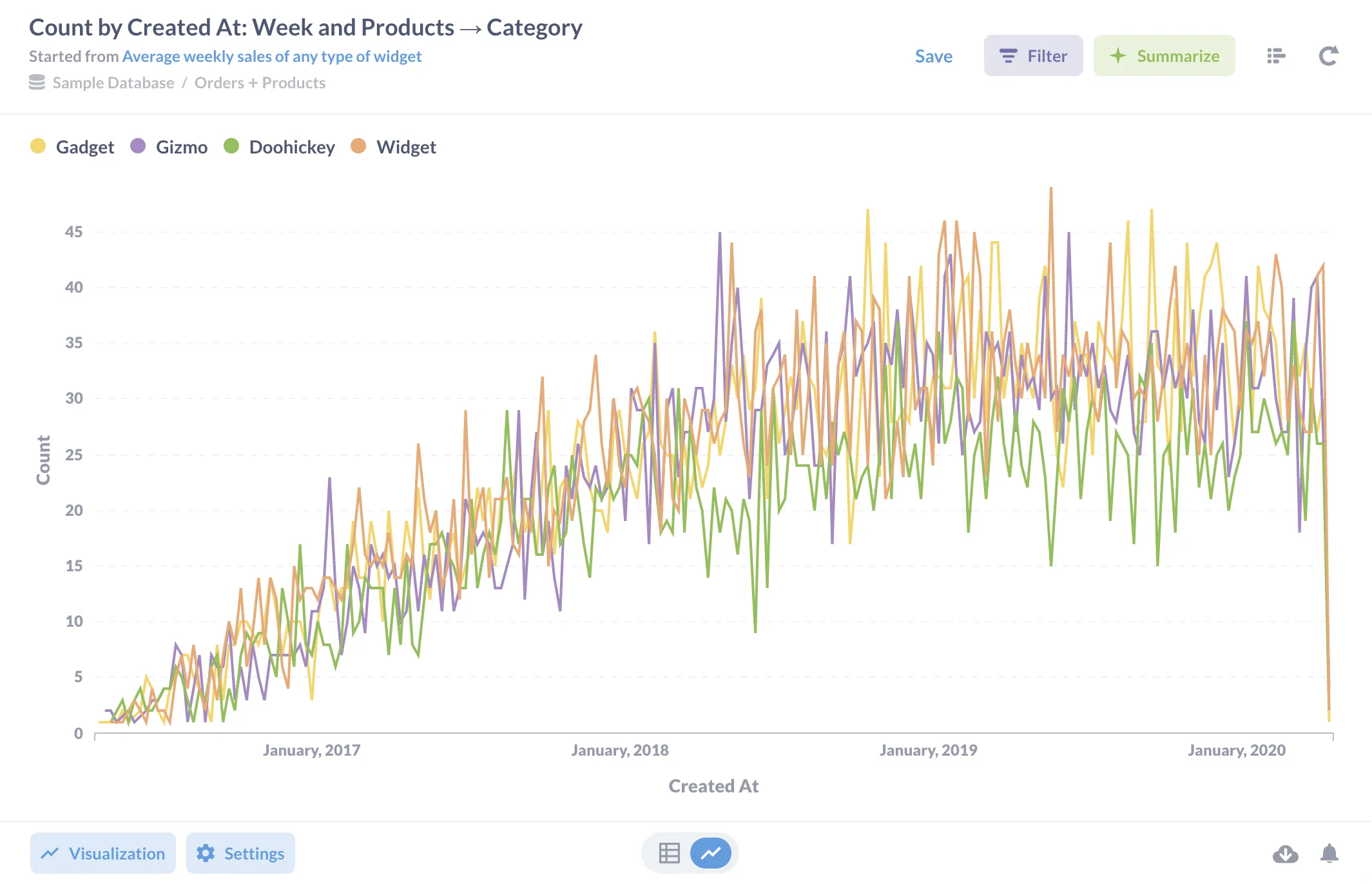Fig. 3. Visualizing weekly totals by category.

However, let’s go further and add another filter after the summary step to take widgets out of our data. When we click the “Filter” button at the bottom of the editor, it shows us the three columns in the summarized data (figure 4).Fig. 4. Options for filtering the summarized data are displayed automatically.

A few clicks lets us create a filter that removes rows whose category is widget (figure 5).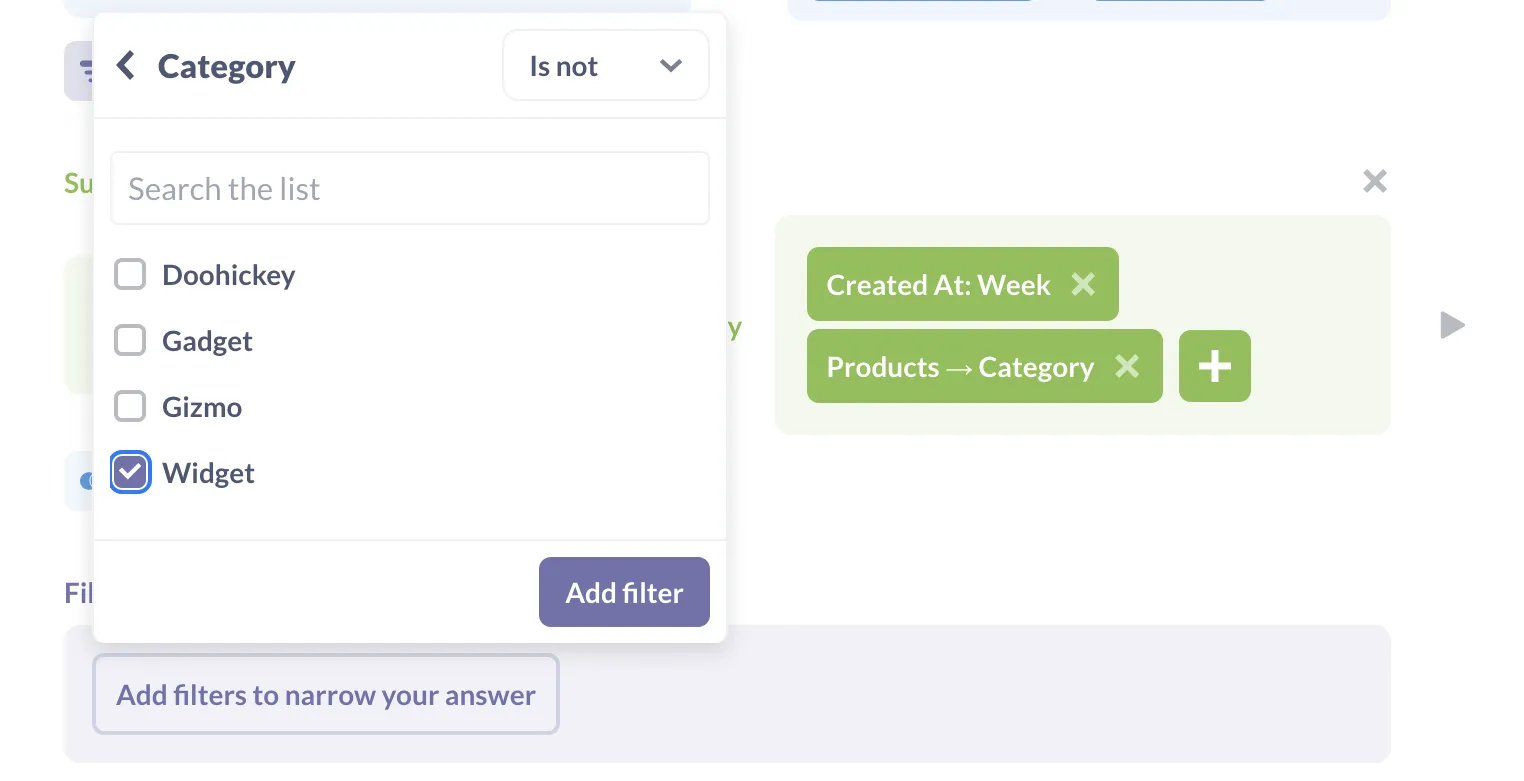Fig. 5. Defining a filter to remove one category from the summary data.

When we save the filter, it’s displayed below the summarization step (figure 6).Fig. 6. Steps in the analysis are displayed in order from top to bottom.

If we visualize the data now, the chart only shows lines for the three product categories we are interested in (figure 7).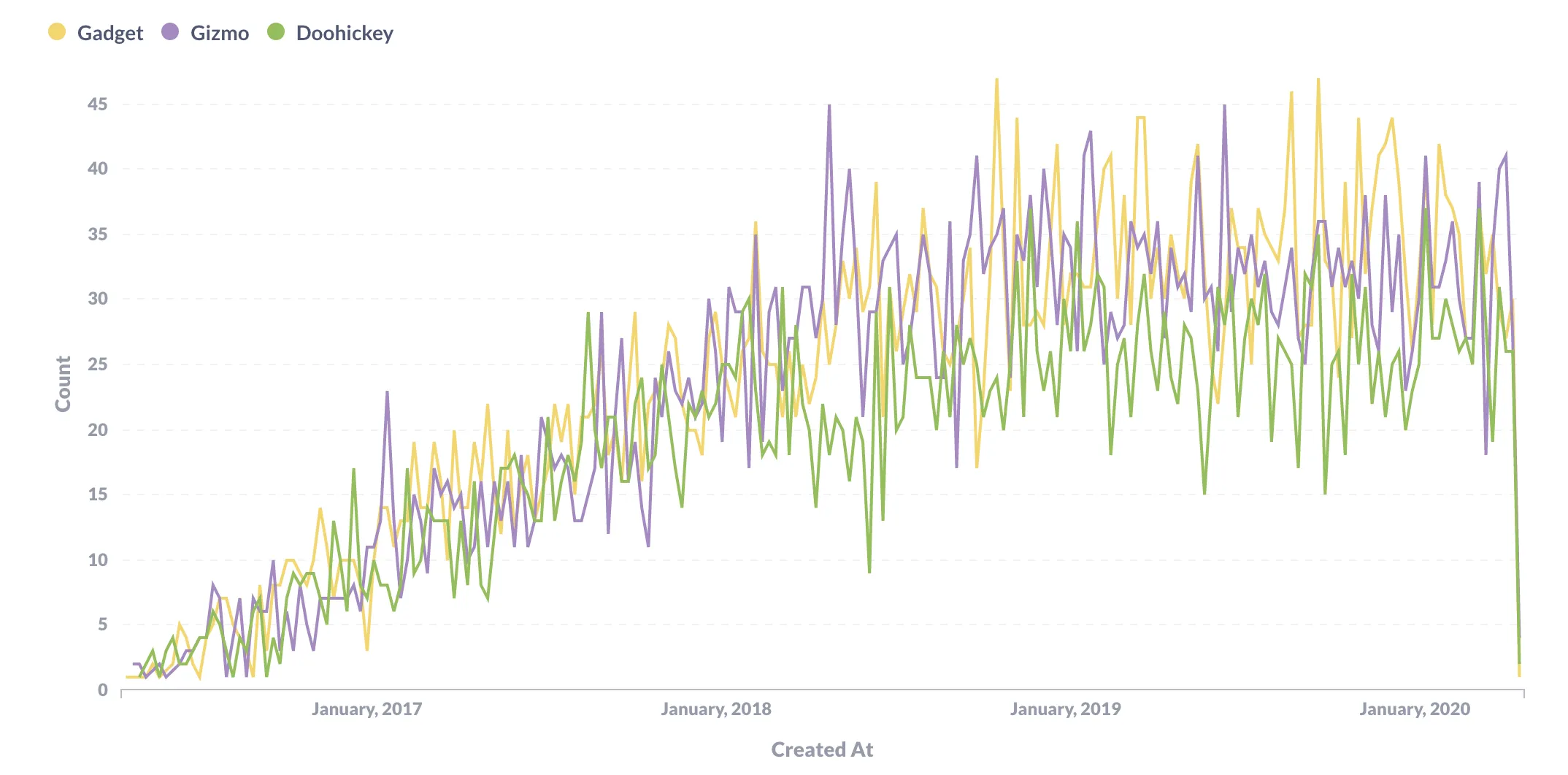Fig. 7: Visualizing the filtered weekliy totals by category.

Now suppose we want to know the average number of widgets of each of these three categories were sold weekly. We have already calculated the weekly sales by category, so all we have to do is click on Summarize, choose Average, and then tell Metabase that we want to average the `Count` grouped by `Category` (figure 8).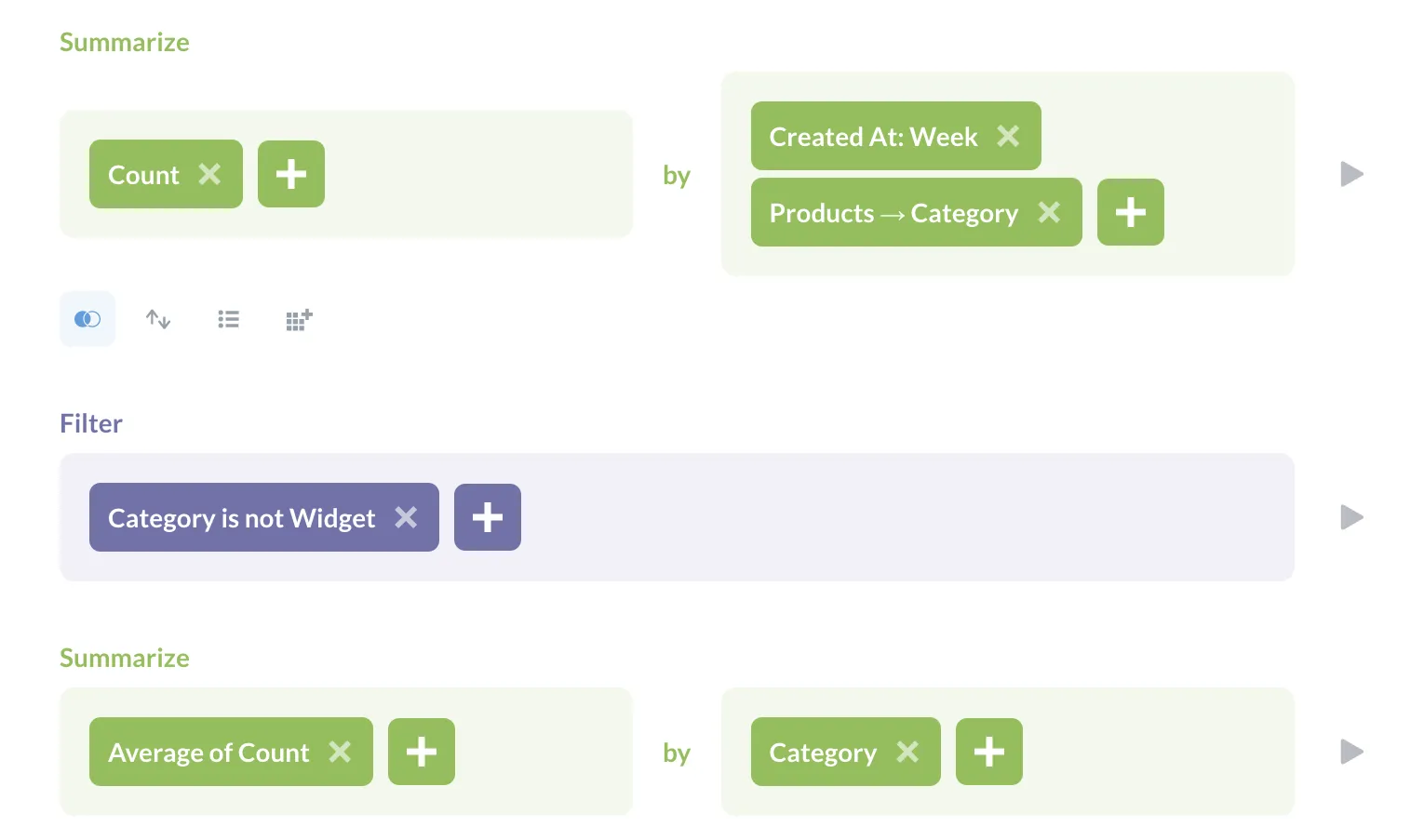Fig. 8. Adding a second summarization step to condense the data even further.

Our visualization is now exactly what we want: the average number of doohickeys, gadgets, and gizmos sold each week (figure 9). The analysis that produces this is shown in figure 10.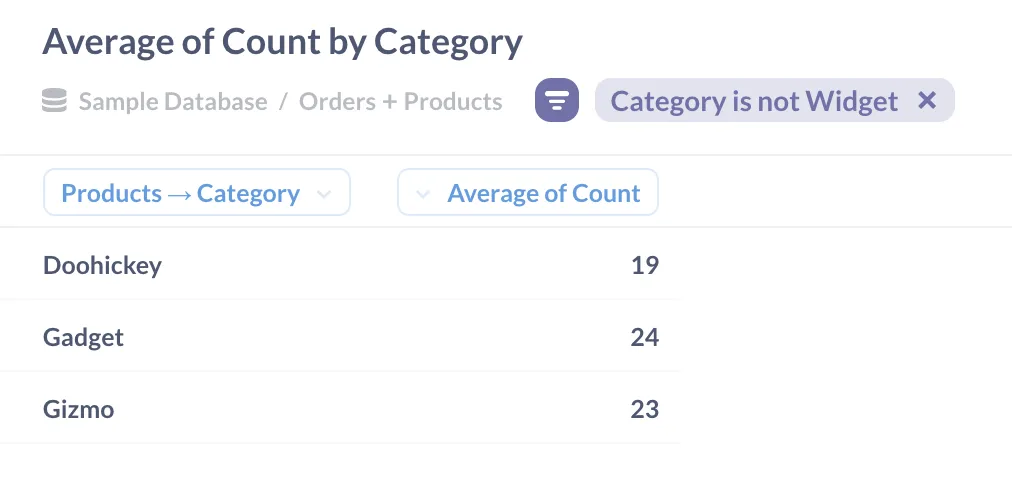Fig. 9. The final summary shows a few key numbers.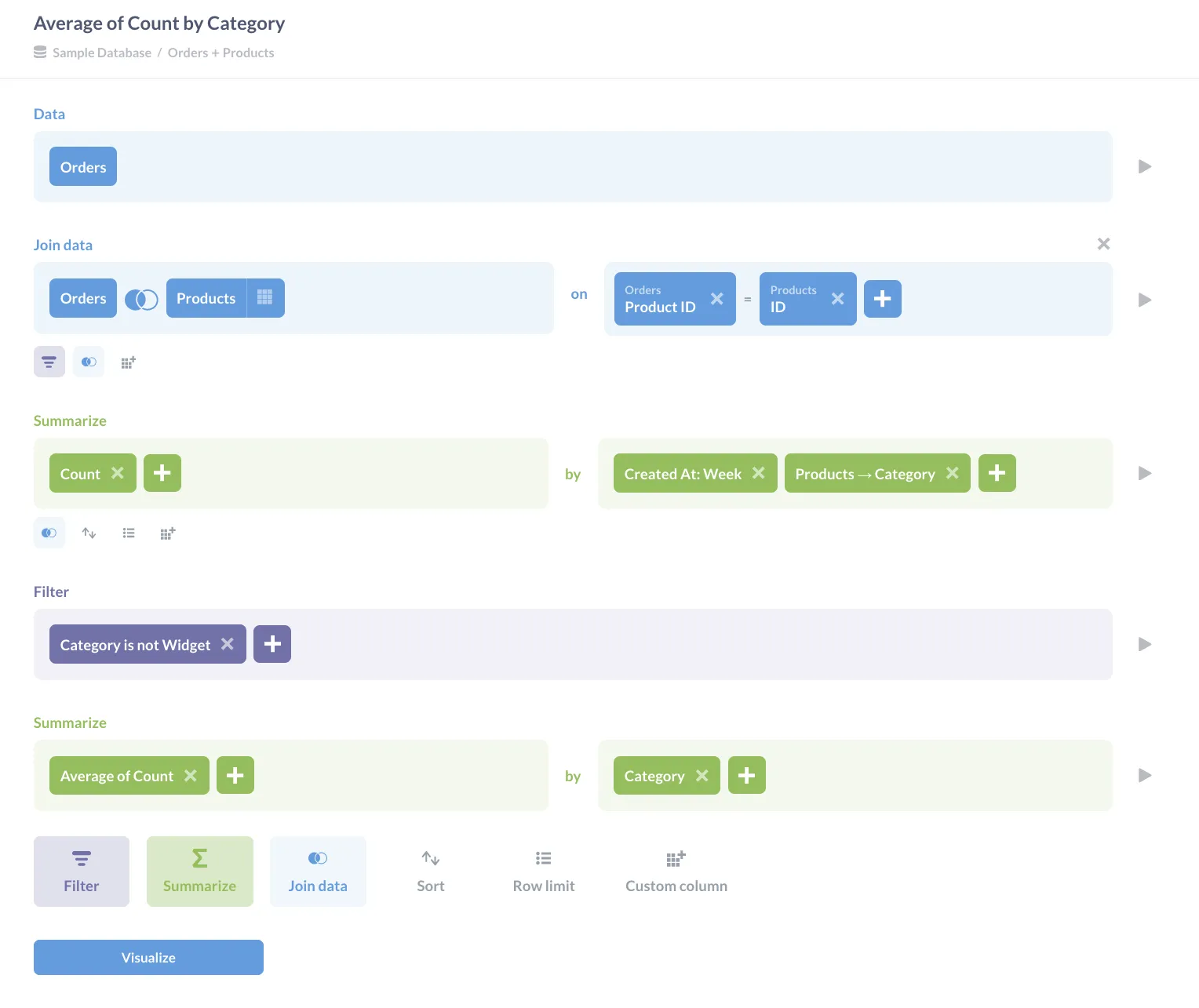Fig. 10. The final analysis shows each step in order from start to finish.

There are other ways to calculate this result: we could, for example, filter out the widgets before we calculated the first summary (which most analysts would consider a best practice, since reducing the size of the data set early on improves performance). The important lesson is that we can construct insight step by step as each answer leads to a new question, and that Metabase allows us to add operations one by one as they occur to us. We don’t have to plan everything in advance: if we find that we are always starting with the same operations, we can save those separately, as explained in the article SQL snippets vs. Saved Questions vs. Views.